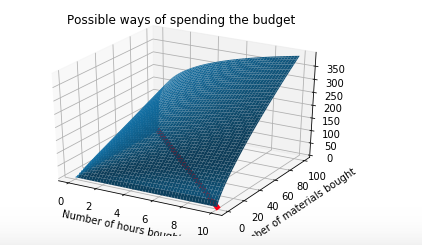# Optimization with constraints using Lagrange Multiplier in Python

Original article was published on Artificial Intelligence on Medium# Optimization with constraints using Lagrange Multiplier in Python

## Lagrange Multiplier on a function with 2 variables with 1 equality constraint

The Lagrange Multiplier is a method for optimizing a function under constraints. In this article, I show how to use the Lagrange Multiplier for optimizing a relatively simple example with two variables and one equality constraint. I use Python for solving a part of the mathematics.

You can follow along with the Python notebook over here.

As an example, let’s take an online marketing department of a company that has received a fixed budget to spent on a mix of Social Media and TV campaigns:

• Suppose we have a fixed budget for spending on marketing of 2500 dollar.
• Suppose we can choose to invest in two types of campaigns: Social Media and TV, where you have to decide
• To simplify, let’s say that one campaign on social media costs 25 dollar and one campaign on TV costs 250 dollar.
• Suppose we have experimented a lot in the past and that we have been able to define the Revenues as a function of the two types of media investments.
• In this notebook, I will show how to find the maximum revenue and the number of different types of campaigns you should buy using Lagrange Multiplier.

## Inspecting the costs

The equation for costs is:

25 dollars times the number of social campaigns + 250 times the number of TV campaigns

Since we want to spend exactly the budget we know that this is equal to 2500 dollar, giving the following constraint:

25 * social + 250 * tv = 2500